# Real number

In mathematics, a real number is a number that can be used to measure a continuous one-dimensional quantity such as a distance, duration or temperature. Here, continuous means that values can have arbitrarily small variations. Every real number can be almost uniquely represented by an infinite decimal expansion. The real numbers are fundamental in calculus (and more generally in all mathematics), in particular by their role in the classical definitions of limits, continuity and derivatives. The set of real numbers is denoted R or and is sometimes called "the reals".The adjective real in this context was introduced in the 17th century by René Descartes to distinguish real numbers, associated with physical reality, from imaginary numbers (such as the square roots of −1), which seemed like a theoretical contrivance unrelated to physical reality. The real numbers include the rational numbers, such as the integer −5 and the fraction 4 / 3. The rest of the real numbers are called irrational numbers, and include algebraic numbers (such as the square root √2 = 1.414...) and transcendental numbers (such as π = 3.1415...). Real numbers can be thought of as all points on an infinitely long line called the number line or real line, where the points corresponding to integers (..., −2, −1, 0, 1, 2, ...) are equally spaced. Conversely, analytic geometry is the association of points on lines (especially axis lines) to real numbers such that geometric displacements are proportional to differences between corresponding numbers. The informal descriptions above of the real numbers are not sufficient for ensuring the correctness of proofs of theorems involving real numbers. The realization that a better definition was needed, and the elaboration of such a definition was a major development of 19th-century mathematics and is the foundation of real analysis, the study of real functions and real-valued sequences. A current axiomatic definition is that real numbers form the unique (up to an isomorphism) Dedekind-complete ordered field. Other common definitions of real numbers include equivalence classes of Cauchy sequences (of rational numbers), Dedekind cuts, and infinite decimal representations. All these definitions satisfy the axiomatic definition and are thus equivalent. (Wikipedia).What are Real Numbers? | Don't Memorise

Watch this video to understand what Real Numbers are! To access all videos on Real Numbers, please enroll in our full course here - https://infinitylearn.com/microcourses?utm_source=youtube&utm_medium=Soical&utm_campaign=DM&utm_content=3YwrcJxEbZw&utm_term=%7Bkeyword%7D In this video, w

From playlist Real NumbersWhat are imaginary numbers?

Imaginary numbers are any numbers that include the imaginary number i. A mix of imaginary and real numbers gives you what’s called a complex number. The primary reason we use imaginary numbers is to give us a way to find the root (radical) of a negative number. There’s no way to use real

From playlist Popular Questions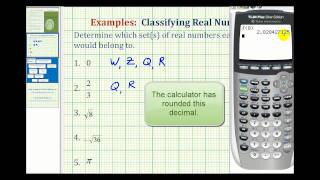Identifying Sets of Real Numbers

This video provides several examples of identifying the sets a real number belongs to. Complete Video Library: http://www.mathispower4u.com Search by Topic: http://www.mathispower4u.wordpress.com

From playlist Number Sense - Properties of Real Numbers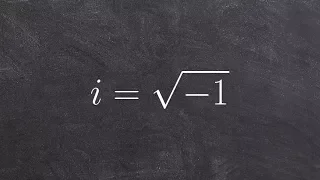Tutorial - What is an imaginary number

http://www.freemathvideos.com In this video playlist you will learn everything you need to know with complex and imaginary numbers

From playlist Complex Numbers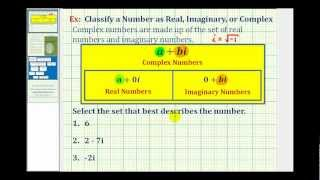Ex: Determine a Real, Imaginary, and Complex Number

This video explains how decide if a number is best described by the set of real, imaginary, or complex numbers. Library: http://mathispower4u.com Search: http://mathispower4u.wordpress.com

From playlist Performing Operations with Complex Numbers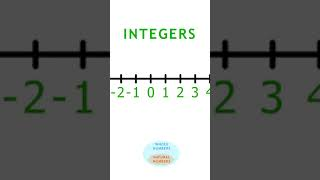Different Types of Numbers on the number line, lesson 1 #shorts

Watch the full playlist: https://www.youtube.com/watch?v=kcxK3_sROZA&list=PL14bv5vXK2WWuODhGbpPQA0GamV5ohOVb&index=1 Natural Numbers (N), (also called positive integers, counting numbers, or natural numbers); They are the numbers {1, 2, 3, 4, 5, …} Whole Numbers (W). This is the set of na

From playlist Celebrities Teach Math: The Number System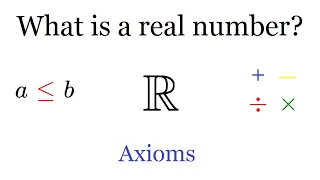What is a real number?

Ordered Fields In this video, I define the notion of an order (or inequality) and then define the concept of an ordered field, and use this to give a definition of R using axioms. Actual Construction of R (with cuts): https://youtu.be/ZWRnZhYv0G0 COOL Construction of R (with sequences)

From playlist Real Numbers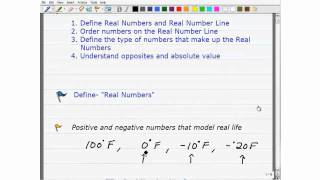Real Number System

http://www.tabletclass.com explains the real number system

From playlist Pre-Algebra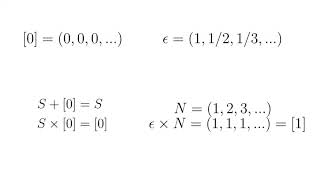Pascal's wager and real numbers

My entry for 3blue1brown's contest, talking about Pascal's wager and how it leads to interesting questions about (hyper)real numbers. A big shoutout to Grant for coming up with this wonderful idea. Link to Thierry Platinis channel for more on hyperreal numbers: https://www.youtube.com/cha

From playlist Summer of Math Exposition Youtube Videos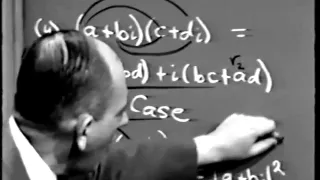Part I: Complex Variables, Lec 1: The Complex Numbers

Part I: Complex Variables, Lecture 1: The Complex Numbers Instructor: Herbert Gross View the complete course: http://ocw.mit.edu/RES18-008F11 License: Creative Commons BY-NC-SA More information at http://ocw.mit.edu/terms More courses at http://ocw.mit.edu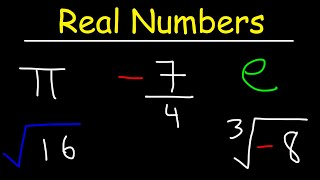Real Numbers

This video provides a basic introduction into real numbers. It explains how to distinguish them from imaginary numbers. It also discusses the difference between rational and irrational numbers as well as integers, natural numbers, and whole numbers. Examples include repeating and non-re

From playlist New Algebra Playlist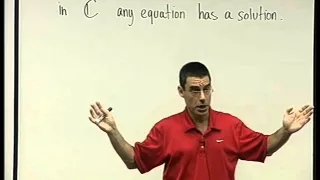05 - Complex numbers

Algebra 1M - international Course no. 104016 Dr. Aviv Censor Technion - International school of engineering

From playlist Algebra 1M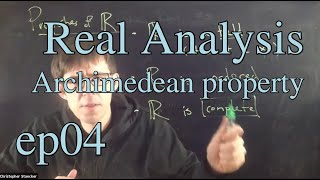Real Analysis Ep 4: The Archimedean Property

Episode 4 of my videos for my undergraduate Real Analysis course at Fairfield University. This is a recording of a live class. This episode is about the Archimedean property of the real numbers. Class webpage: http://cstaecker.fairfield.edu/~cstaecker/courses/2020f3371/ Chris Staecker w

From playlist Math 3371 (Real analysis) Fall 2020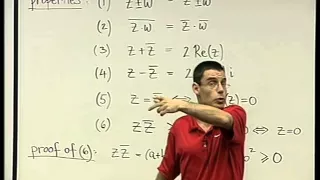06 - The Complex conjugate, the modulus and division

Algebra 1M - international Course no. 104016 Dr. Aviv Censor Technion - International school of engineering

From playlist Algebra 1M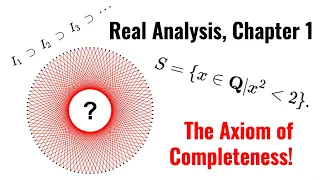Real Analysis Chapter 1: The Axiom of Completeness

Welcome to the next part of my series on Real Analysis! Today we're covering the Axiom of Completeness, which is what opens the door for us to explore the wonderful world of the real number line, as it distinguishes the set of real numbers from that of the rational numbers. It allows us

From playlist Real Analysis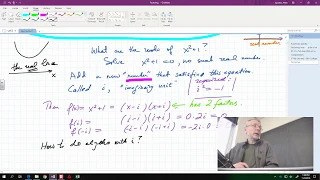Complex numbers -- College Algebra

This lecture is on College Algebra. It follows the introductory part of the book Calculus Illustrated by Peter Saveliev. The text of the book can be found at http://calculus123.com.

From playlist College Algebra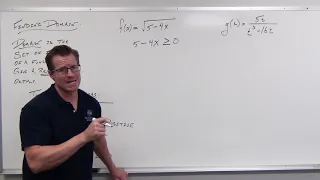Finding the Domain of Functions (Precalculus - College Algebra 4)

Support: https://www.patreon.com/ProfessorLeonard Cool Mathy Merch: https://professor-leonard.myshopify.com/ What the Domain means for functions, what to look for, and how to find it for basic functions.

From playlist Precalculus - College Algebra/Trigonometry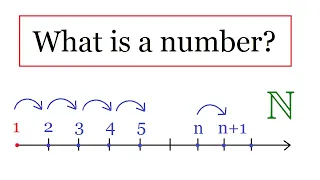What is a number ?

Construction of Natural Numbers In this, I rigorously define the concept of a natural number, using Peano's axioms. I also explain why those axioms are the basis for the principle of mathematical induction. Enjoy! Check out my Real Numbers Playlist: https://www.youtube.com/playlist?list=

From playlist Real Numbers